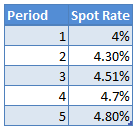# How to Price a Bond Using Spot Rates (Zero Curve)

The simplest way to calculate the value of a bond is to take the cash flows of the bond till its maturity and then discount them by a single discount rate. The method is quick but not very accurate because the yield curve is not flat and the interest rates are different for different maturities. A better way to price the bonds is to discount each cash flow with the spot rate (zero coupon rate) for its respective maturity.

Example 1

Let’s take an example. Suppose we want to calculate the value of a $1000 par, 5% coupon, 5 year maturity bond. We also have the following spot rates for the next 5 years:Assuming this is an annual pay bond, the bond will have the following cash flows. Year 1:$50

Year 2: $50 Year 3:$50

Year 4: $50 Year 5:$1000 + $50 The value of the bond can be calculated by discounting these cash flows by their respective spot rate. Bond Value = 50/(1.04)^1+50/(1.0430)^2+50/(1.0451)^3+50/(1.047)^4+1050/(1.048)^5 Bond Value =$1010.033

Example 2

Let’s take another example. Suppose we have a bond that matures in 2 years, that has a coupon rate of 6%, and pays coupon semi-annually. The spot rates are 3.9% for 6 months, 4% for 1 year, 4.15% for 1.5 years, and 4.3% for 2 years.

The cash flows from this bond are $30,$30, $30, and$1030.

The value of the bond will be calculated as follows:

Bond value = $30/(1+3.9%/2)^1+$30/(1+4%/2)^2+$30/(1+4.15%/2)^3+$1030/(1+4.3%/2)^4

Bond value = \$1032.45

You can use the above formula to value any bond with any maturity. All you need is the spot rate for the respective maturity. Since spot rates are bootstrapped from yield curve, we may have to interpolate yields for certain maturities for which Treasury securities are not available.